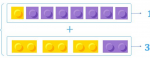Smartick is an online platform for children to master math in only 15 minutes a day

# I want to learn about: Number & Operations – Fractions

Content about fractions in elementary mathematics. Exercises, tutorials, problems, and teaching resources for fractions in elementary. Here you will find posts about fractions to make learning mathematics easier and more fun. ido generado sobre fracciones de matemáticas de primaria.

Dec01

## Using Mixed Numbers to Represent Improper Fractions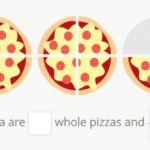In today’s post we’re going to learn about mixed numbers, which are much easier to interpret than the improper fractions they represent. We’re going to look at some examples of the exercises and problems that you can work on in Smartick and the types of numbers that you can work on in these activities. You’ll be able to do them […]

Nov20

## Singapore Bar Model and PercentagesIn today’s post, we’re going to look at percentages through the Singapore bar model. To define a part of a whole, we can use a fraction, but we can also use a percentage. The whole divides into 100 equal parts, therefore each part is 1/100 of the whole or 1%.  Let’s look at an example of […]

Nov06

## Understanding the Division of Fractions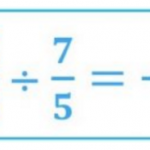Today we’re going to learn how to perform a division of fractions using the very definition of division as the inverse operation of multiplication. This way we don’t need to use the more known methods that we’ve already seen in the post about Dividing fractions. You might know how to use those methods, but without knowing […]

Oct18

## Adding Fractions with the Visual Aid of Rectangles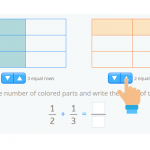In today’s post, we’re going to look at an example of how to add fractions. Fractions might seem boring, but, just like anything mathematical, once you understand them they become easy and fun! To help us explain adding fractions, we’re going to use a resource that we’ve recently developed for the Smartick platform. We think you’ll find […]

Oct04

## Fraction Word Problems: Addition, Subtraction, and Mixed NumbersIn today’s post, we’re going to see how to solve some of the new problems that we’ve introduced in Smartick, fraction word problems. They appear during the word problems at the end of the daily session. We’re going to look at how to solve problems involving addition and subtraction of fractions, including mixed fractions (the […]

Sep06

## Solve Fraction Problems with Halves, Thirds and QuartersVery often we find, both in mathematical problems and in real life, fraction phrases like: a half, a third or a quarter. They are the most famous fractions! I’m sure you’ve seen them before. If you want to see what they are and how to calculate them, you can check out this link: Calculating halves, thirds […]

Sep04

## Learn How to Solve Fractions with an Unknown VariableIn this post, we are going to learn to solve one of the situations in which we could encounter fractions with an unknown variable. First, you should review what equivalent fractions are in some of our previous posts: Concept of equivalent fractions Examples of equivalent fractions We could encounter a mathematic expression with an unknown […]

Aug21

## What Is a Fraction? Definition and Various MeaningsWhat’s a fraction? It’s not an easy question to answer. Despite it being such a familiar element, fractions have various meanings, which makes it tricky to define and in turn, teach, and understand. Fractions can be interpreted in no less than five ways; we’ll look at those ways in this post. The different meanings of […]

Aug16

## Review Factoring with Examples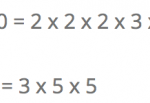Hello again! Do you know what factoring is? Do you know what it is used for? In this post we will answer these questions. How to Factor The factorization of a number is performed by writing the number as the multiplication of all its prime factors. Example: 12 = 2 x 2 x 3 You can […]

Jul26

## Learn How to Subtract Fractions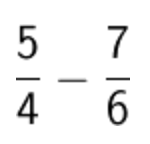In today’s post, we are going to look at how to subtract fractions; but in order subtract them, first you’ll have to know what the denominator and numerator mean in a fraction: The numerator is the number that is written in the top part of the fraction and the denominator is the number that is […]

Jul14

## Learn How to Divide Fractions with Two Different Methods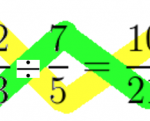In this post, we are going to learn how to divide fractions. In order to do so, I’m going to teach you two simpler ways that will help you divide fractions.   Method 1 to Divide Fractions: Cross Multiplication This method consists of multiplying the first fraction’s numerator by the second fraction’s denominator. Then we place that […]